# A basketball is dribbled down a basketball court by Cephan Sturry at a velocity of 2.00 m/s. At the moment it is shot to...

A basketball is dribbled down a basketball court by Cephan Sturry at a velocity of 2.00 m/s. At the moment it is shot towards the hoop, the player is 7.24m from the hoop, and that hoop is 3.00m from the ground. The angle between the ball and the hoop is 65o when it is shot. If the basketball rotates from the moment of release until it reaches the hoop at 2 rotations per second, how many rotations will have been made until the ball reaches the hoop?

The statement is not clear. What is the meaning of angle between the ball and hoop? They probably mean the angle between the line joining the hoop to launch point and the velocity vector of ball. Then the total launch angle would beTotal time to reach the hoopNo.of rotations in this time,##### Add Answer of: A basketball is dribbled down a basketball court by Cephan Sturry at a velocity of 2.00 m/s. At the moment it is shot to...
Similar Homework Help Questions
• ### A basketball player scores a three point shot from the three point line, which is 7.24...

A basketball player scores a three point shot from the three point line, which is 7.24 m from the hoop. The ball was thrown from a height of 2.00 m above the court at an angle of 45.0. The hoop is 3.00 m above the court and air resistance can be ignored. (a) What speed was the ball thrown at? (b) How long did the ball take to reach the hoop? (c) What were the velocity components of the ball...

• ### A basketball player attempts a three-point shot 10.0 m from the basket as shown in the...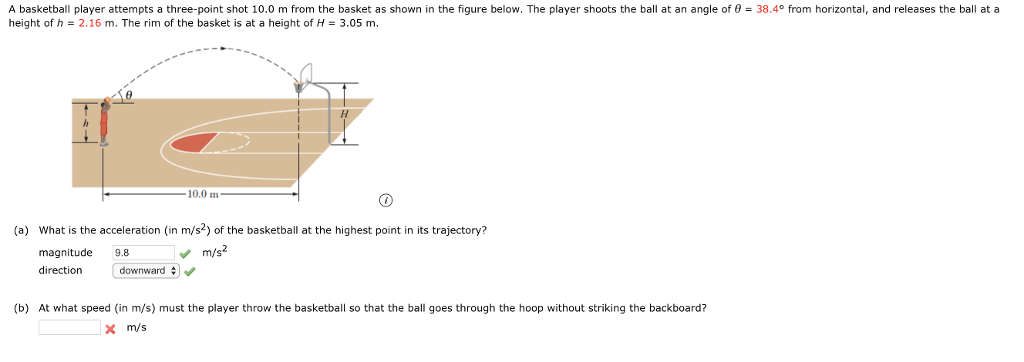A basketball player attempts a three-point shot 10.0 m from the basket as shown in the figure below. The player shoots the ball at an angle of θ = 38.4° from horizontal, and releases the ball at a height of h 2.16 m. The rim of the basket is at a height of H- 3.05 m 10,0 m (a) What is the acceleration (in m/s2) of the basketball at the highest point in its trajectory? m/s2 magnitude 9.8 direction downward...

• ### When a basketball player shoots a foul shot, the ball follows a parabolic arc. This arc...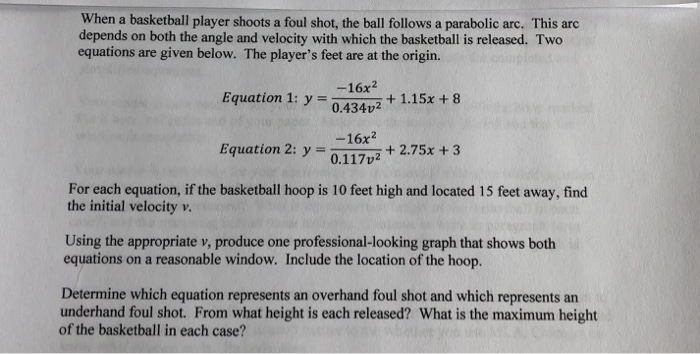When a basketball player shoots a foul shot, the ball follows a parabolic arc. This arc depends on both the angle and velocity with which the basketball is released. Two equations are given below. The player's feet are at the origin. -16x2 Equation 1: y 0.434v2 + 1.15x +8 Equation 2: y72.75x +3 -16x2 0.117v2 For each equation, if the basketball hoop is 10 feet high and located 15 feet away, find the initial velocity v. Using the appropriate v,...

• ### 5) A 2.00 m tall basketball player is standing on the floor exactly 10.0 m from...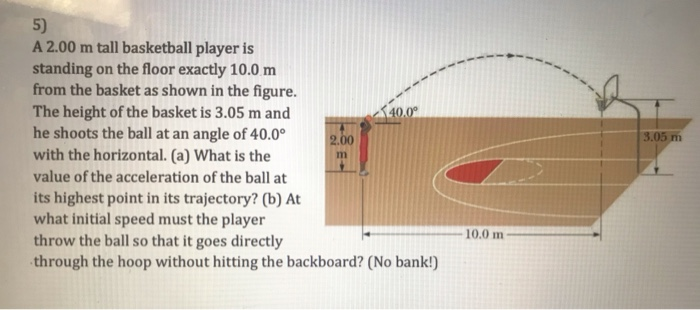5) A 2.00 m tall basketball player is standing on the floor exactly 10.0 m from the basket as shown in the figure. The height of the basket is 3.05 m and he shoots the ball at an angle of 40.0 2.00 with the horizontal. (a) what is the value of the acceleration of the ball at its highest point in its trajectory? (b) At what initial speed must the player throw the ball so that it goes directly through...

• ### A 2.00 m tall basketball player is standing on the floor exactly 10.0 m from the...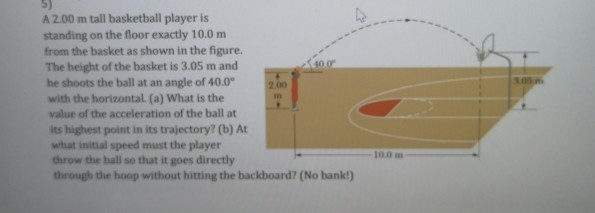A 2.00 m tall basketball player is standing on the floor exactly 10.0 m from the basket as shown in the figure. The height of the basket is 3.05 m and he shoots the ball at an angle of 40.0 with the horizontal. (a) What is the value of the acceleration of the ball at its highest point in its trajectory? (b) At what initial speed must the player throw the ball so that it goes directly 10.0 m through...

• ### In the 1970 National Basketball Association championship, Jerry West made a 60 ft shot from beyond...

In the 1970 National Basketball Association championship, Jerry West made a 60 ft shot from beyond half court to lead the Los Angeles Lakers to an improbable tie at the buzzer with the New York Knicks. Suppose West threw the ball at an angle of 50.0∘ above the horizontal. The basket is 10 ft from the court floor. Neglecting air resistance, estimate the initial speed of the ball. (The Knicks won the game in overtime.) Remember to account for the...

• ### Question 9 (1 point) A basketball player who is 2.00 m tall is standing on the...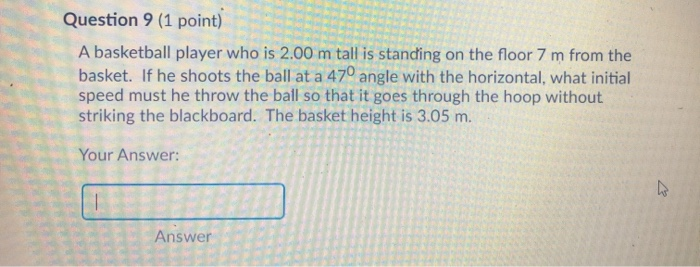Question 9 (1 point) A basketball player who is 2.00 m tall is standing on the floor 7 m from the basket. If he shoots the ball at a 470 angle with the horizontal, what initial speed must he throw the ball so that it goes through the hoop without striking the blackboard. The basket height is 3.05 m. Your Answer: Answer

• ### A basketball is shot from an initial height of 2.40 m (Figure 1)with an initial speed...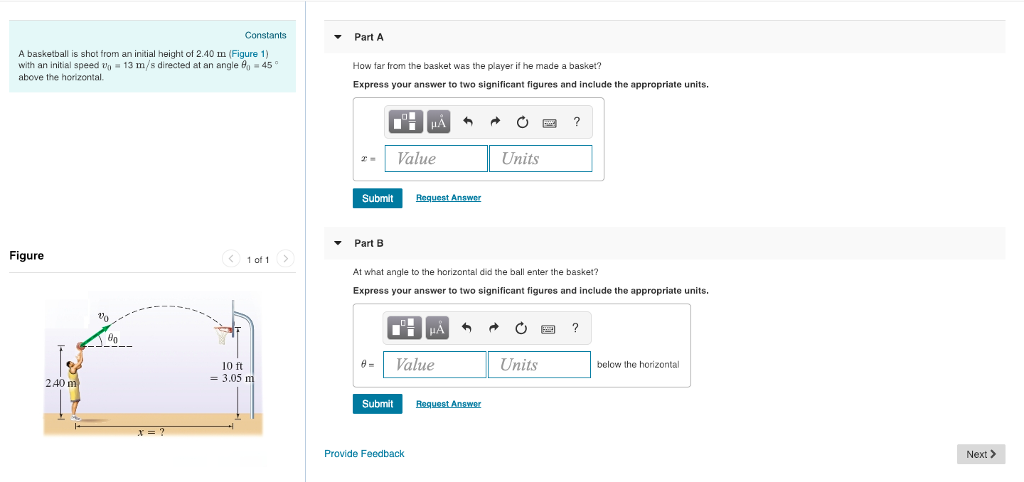A basketball is shot from an initial height of 2.40 m (Figure 1)with an initial speed v0 = 13 m/s directed at an angle θ0 = 45 ∘above the horizontal. A)How far from the basket was the player if he made a basket? B) At what angle to the horizontal did the ball enter the basket?

• ### A 2.00 METER TALL BASKETBALL PLAYER ATTEMPTS A GOAL 10.00 METERS FROM THE BASKET(3.05 METERS HIGH)

A 2.00 METER TALL BASKETBALL PLAYER ATTEMPTS A GOAL 10.00 METERS FROM THE BASKET(3.05 METERS HIGH). IF HE SHOOTS THE BALL AT A 45.0 DEGREE ANGLE, AT WHAT INITIAL SPEED MUST HE THROW THE BALL SO THAT IS GOES THROUGH THE HOOP WITHOUT STRIKING THE BACKBOARD? Initial hi=2.0 final hf=3.05 horizontal distance=10 Here are the equations: hf=Hi + Vsin45*t - 4.9 t^2 and 10= Vcos45*t In the second equation, solve for t in terms of the numbers and V. Then...

• ### 1. In the 1970 National Basketball Association championship, Jerry West made a 60 ft shot from...

1. In the 1970 National Basketball Association championship, Jerry West made a 60 ft shot from beyond half court to lead the Los Angeles Lakers to an improbable tie at the buzzer with the New York Knicks. Suppose West threw the ball at an angle of 45.0∘above the horizontal. The basket is 10 ft from the court floor. Neglecting air resistance, estimate the initial speed of the ball. (The Knicks won the game in overtime.) 2. Robin would like to...

Need Online Homework Help?